# Use SUMIFS function with multiple criteria### Graphic skills

In our work, we often need to filter through multiple criteria for statistics. At this time, we can use the SUMIFS function.

The grammatical structure of the SUMIFS function is: SUMIFSSum range, Range1, Criteria 1, Range2, Criteria2...）”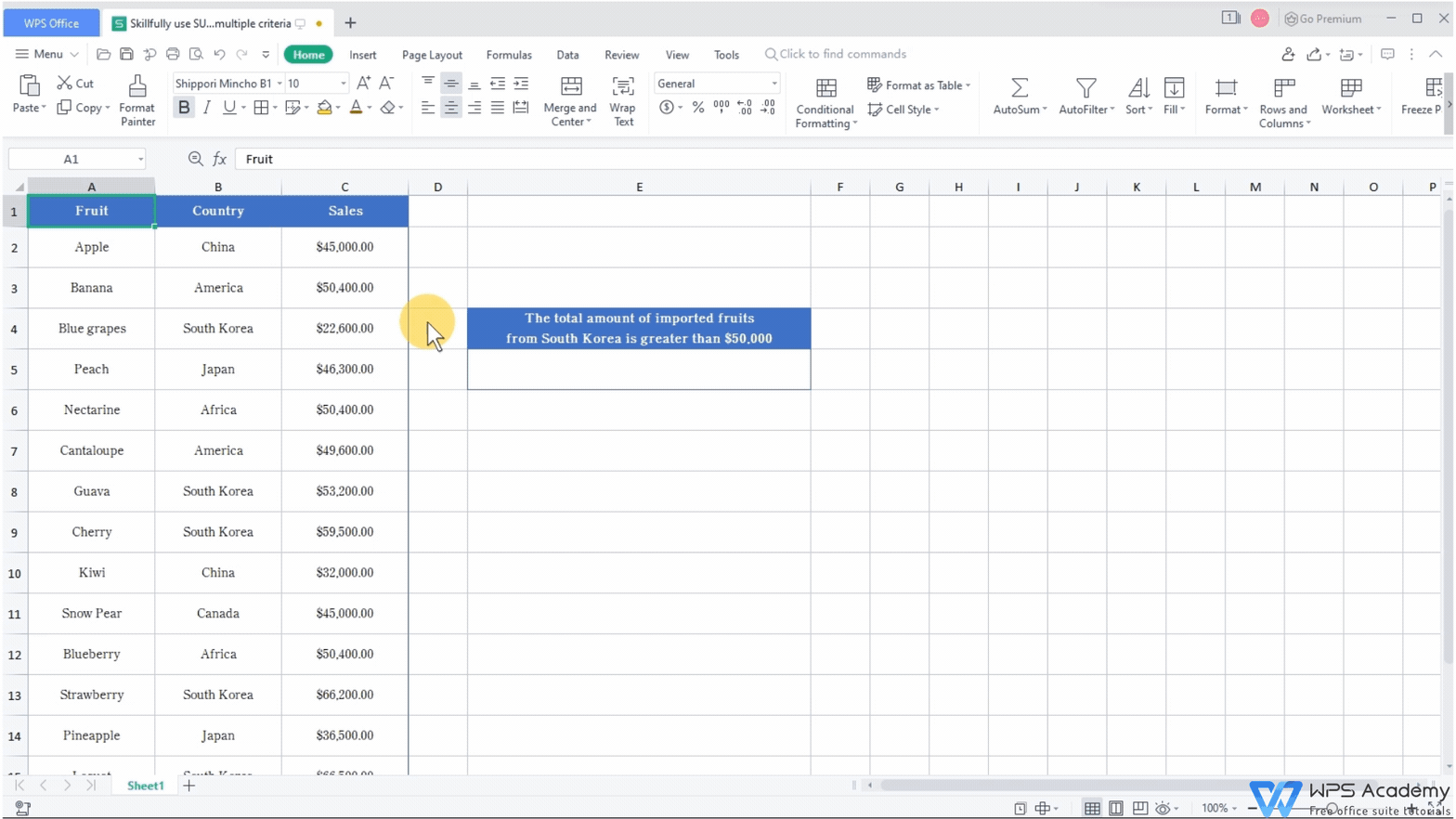Using the data in this table as an example, we want to calculate The total amount of imported fruits from South Korea greater than \$50,000. There are two conditions. One is South Korea, and the other is the amount>\$50,000.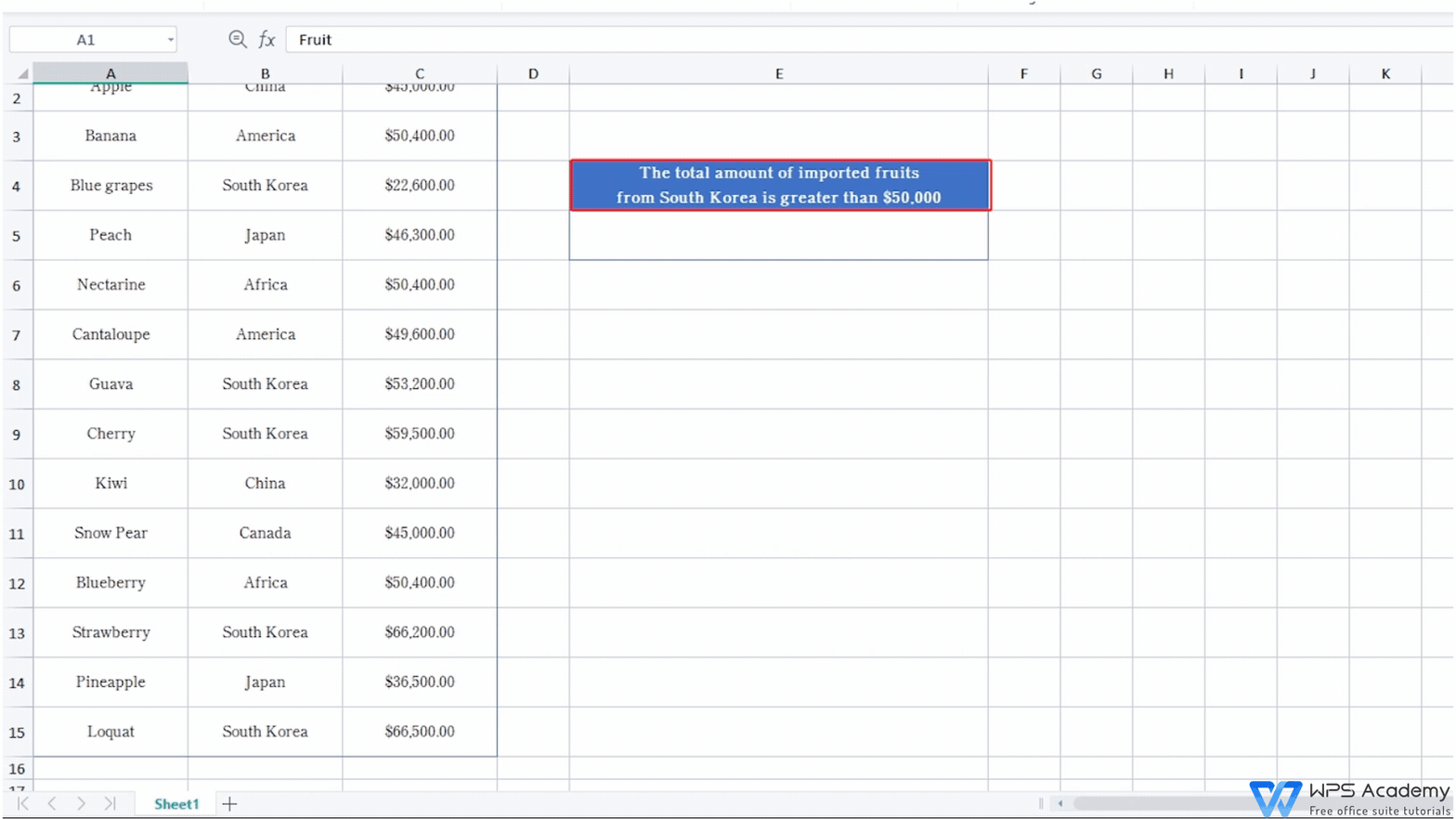First, after clicking cell E5, click Insert Function. After entering SUMIFS in the Search for Function of the dialog box, click the OK button. Then, the Function Arguments dialog box will pop up.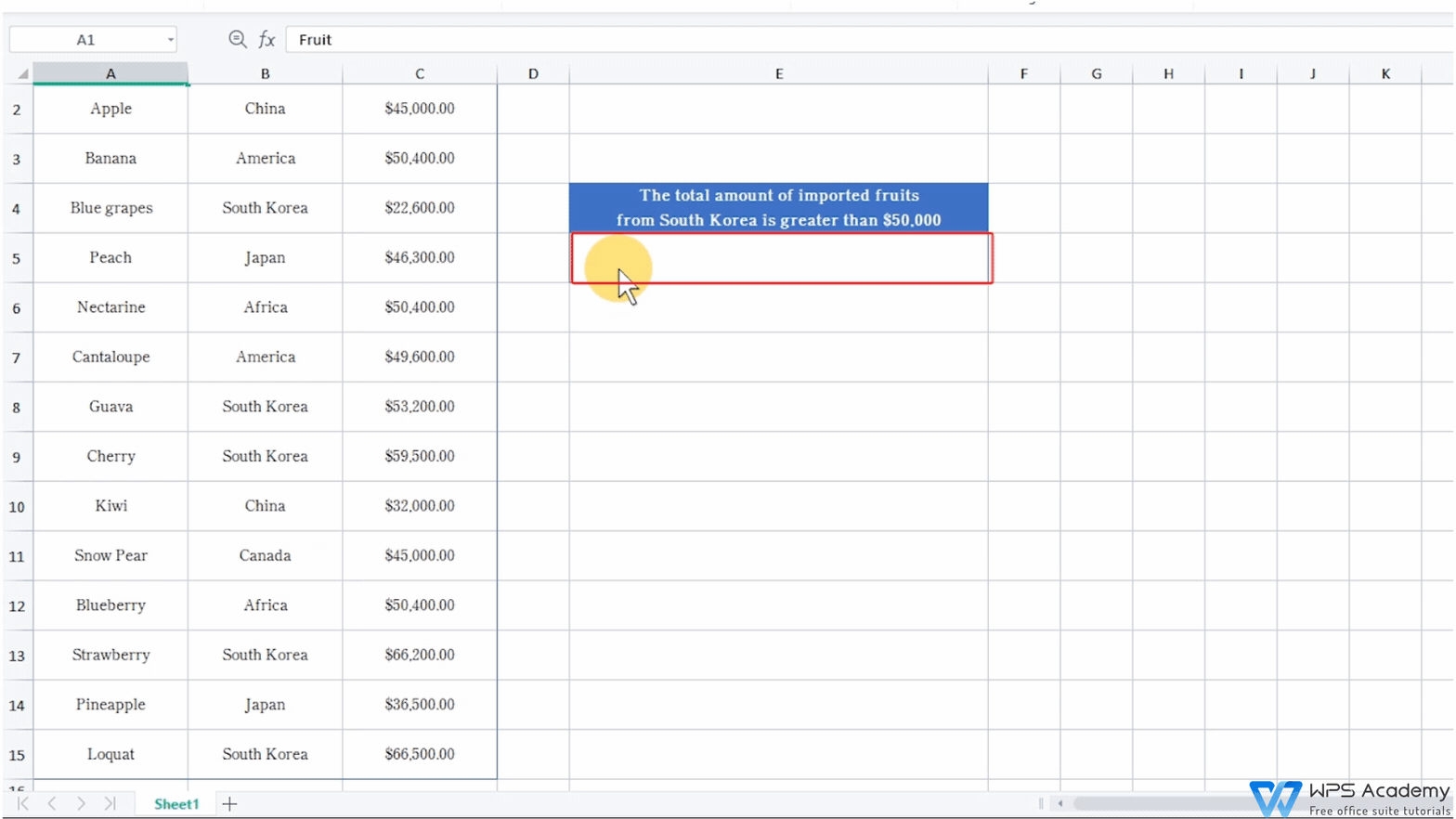Sum range refers to the actual cells used for sum calculation. In this table, we finally need to sum the amounts, so the corresponding sum range is the data amount in the Sales column, namely C2-C15.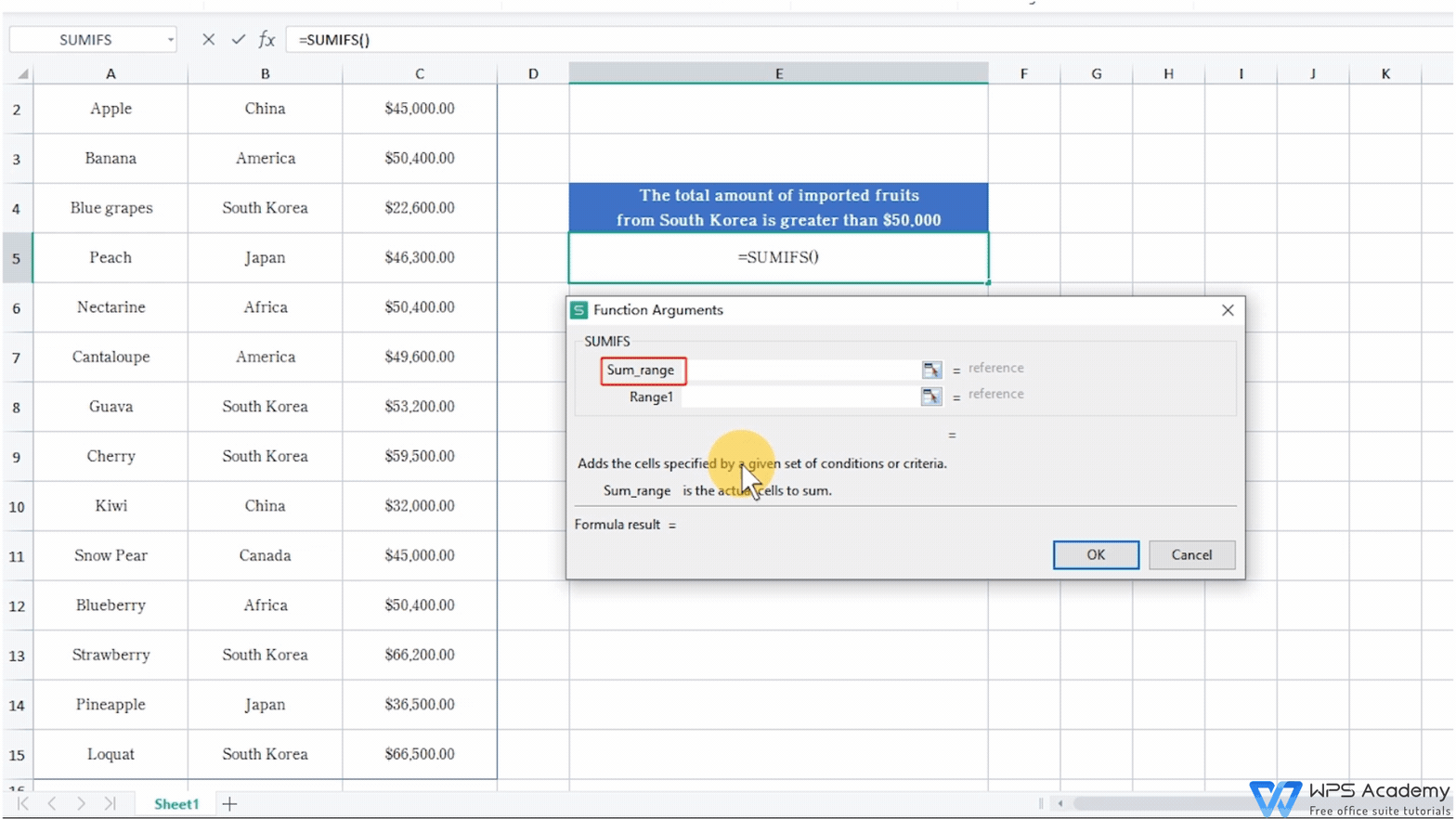Range 1 is the calculation area of Criteria 1. Given that Criteria 1 is South Korea, the corresponding calculation area is the data in the Country column, namely B2-B15.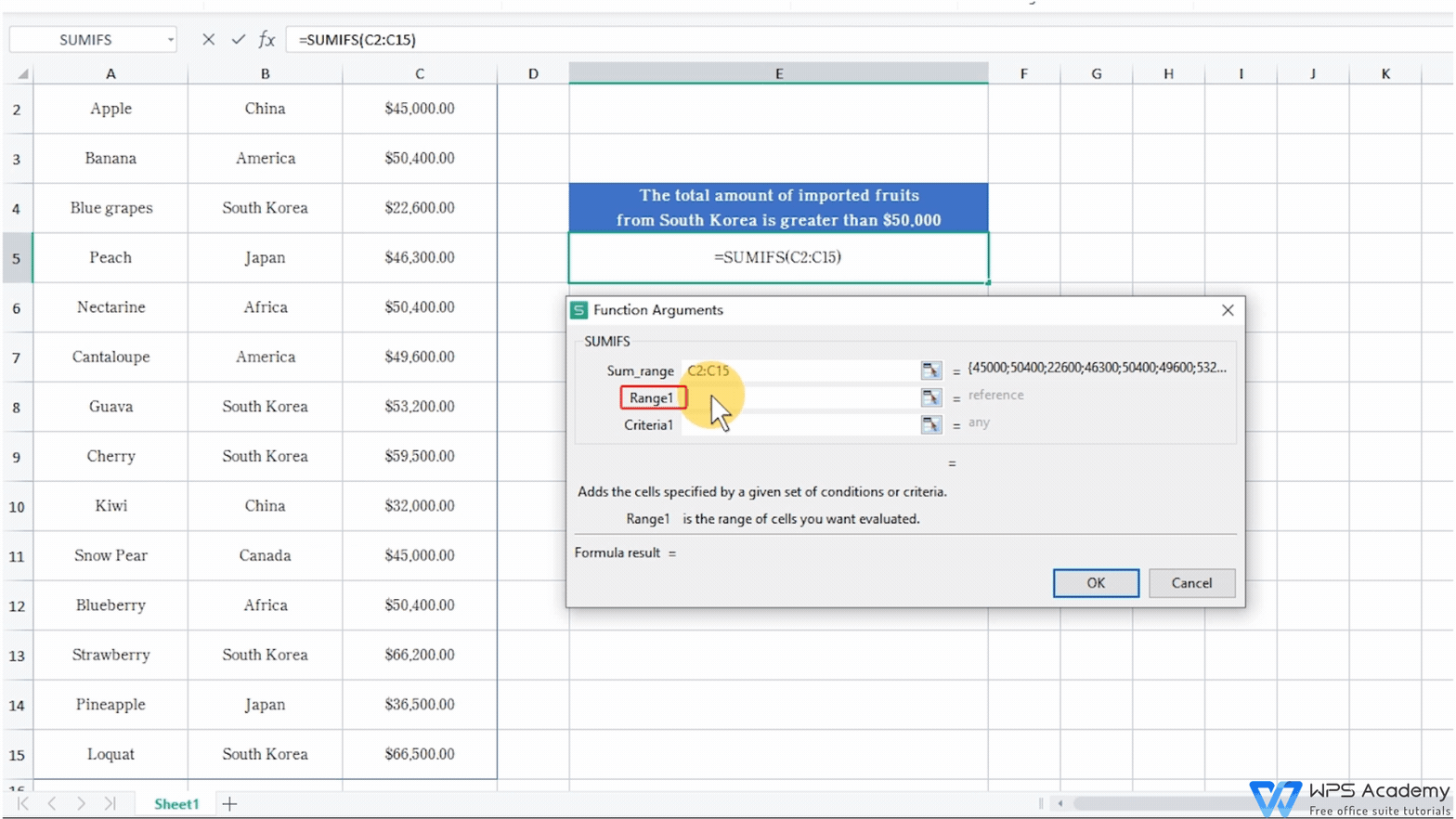Criteria 1 is South Korea, so we enter South Korea here. When entering conditional parameters, we need to add double quotation marks for quotation.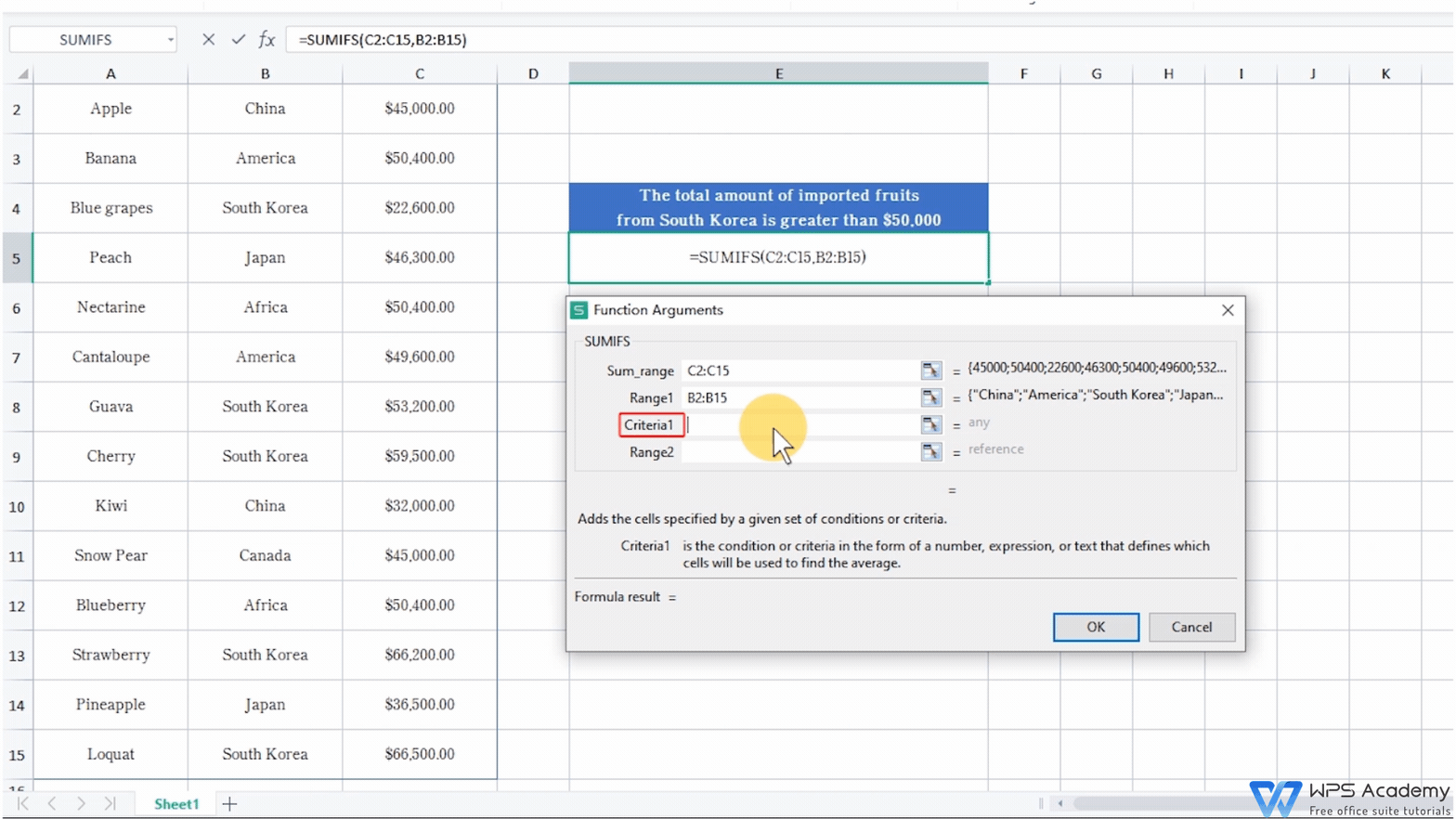We can add the criteria in sequence later, and each additional condition requires a corresponding criteria range for calculation.

Range 2 is the corresponding area for Criteria 2 (the amount>\$50,000), namely the data amount of the area in the table: C2-C15 cell area.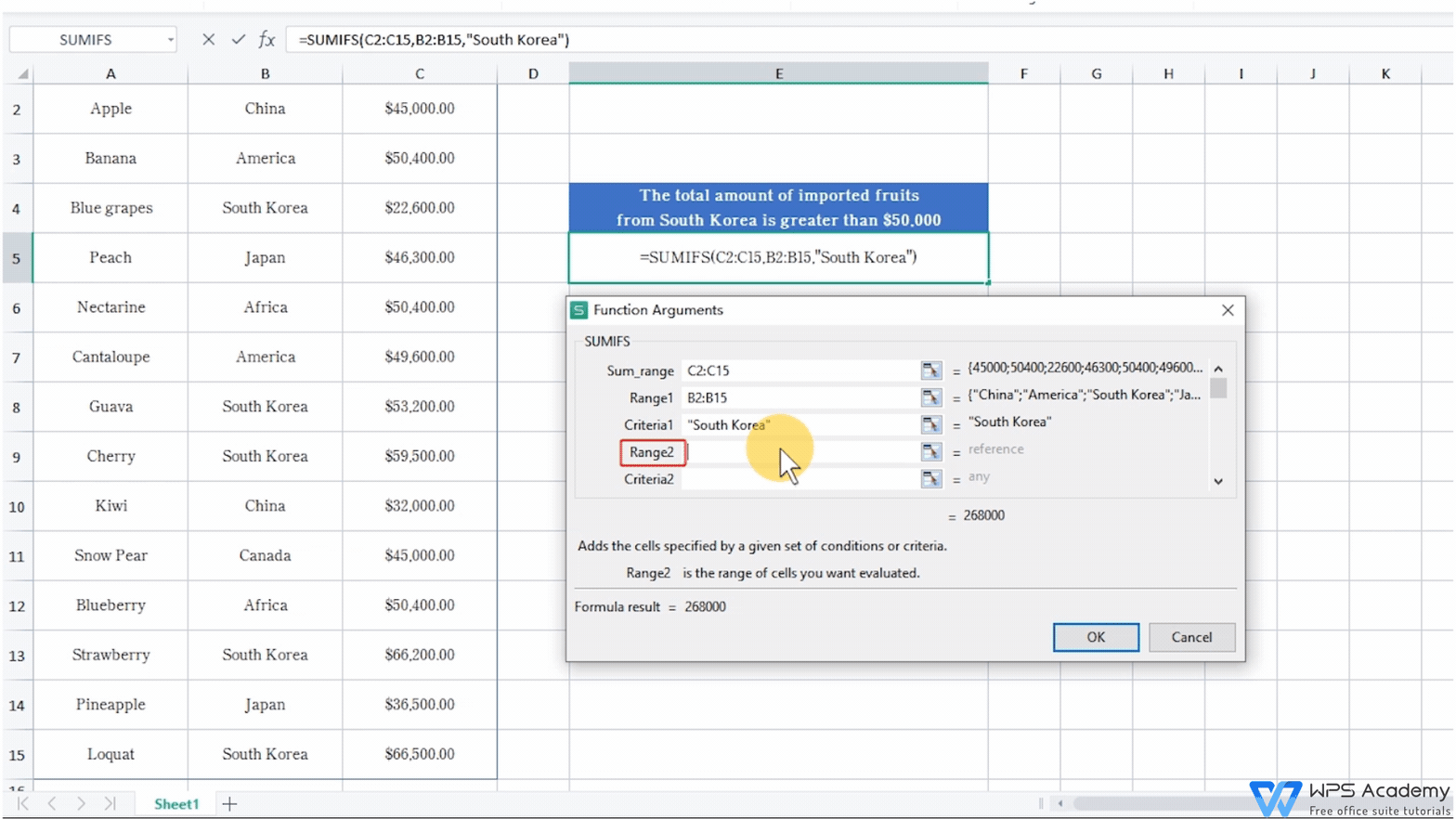Enter >50,000 for Criteria 2 to indicate that the amount is >\$50,000.

Then click the OK button to calculate the total amount of imported fruit from Korea over \$50,000 is \$24,540,000.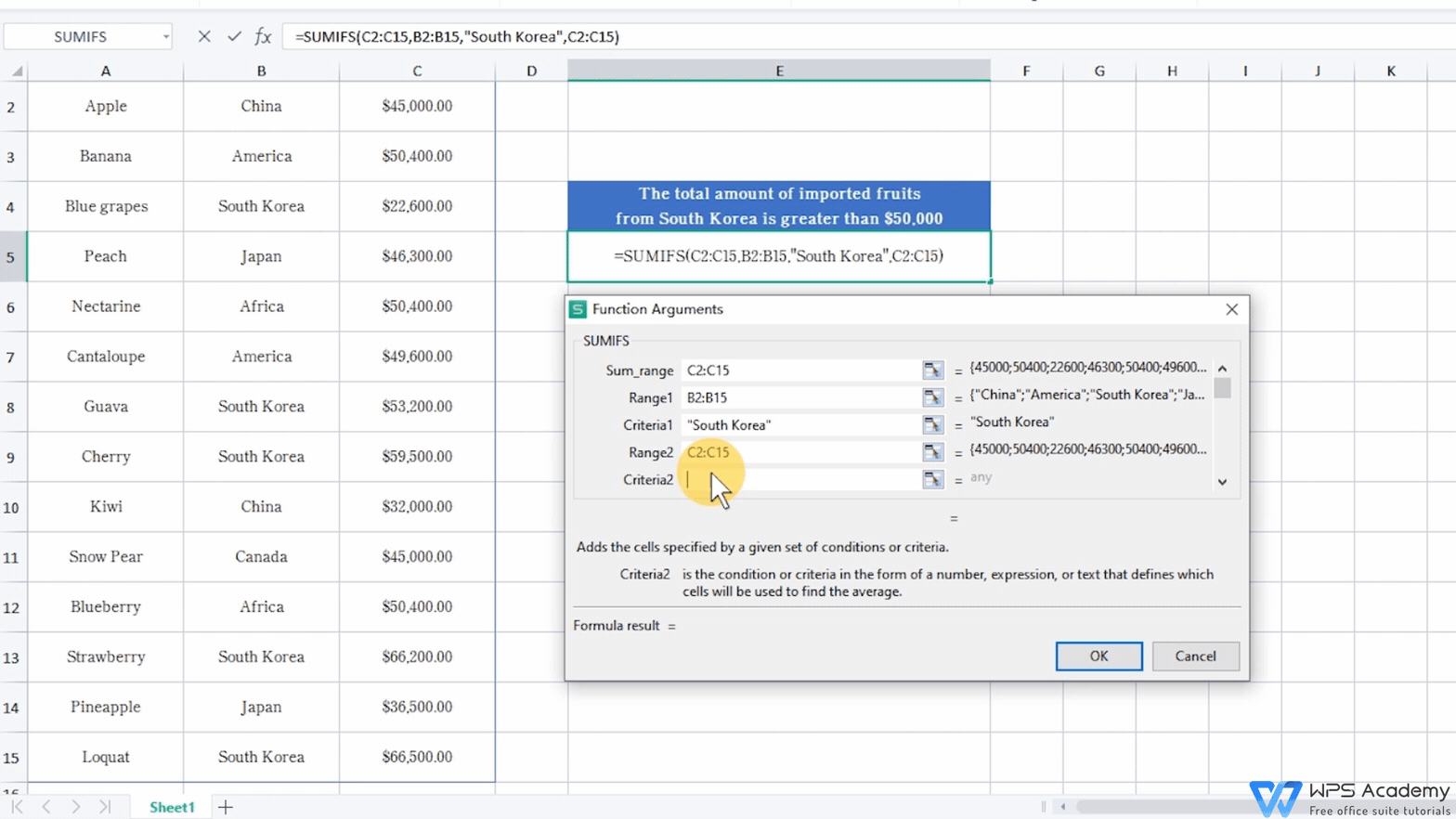This is the basic use of SUMIFS function. Did you get it? For further study, you're welcome to enter WPS Academy.